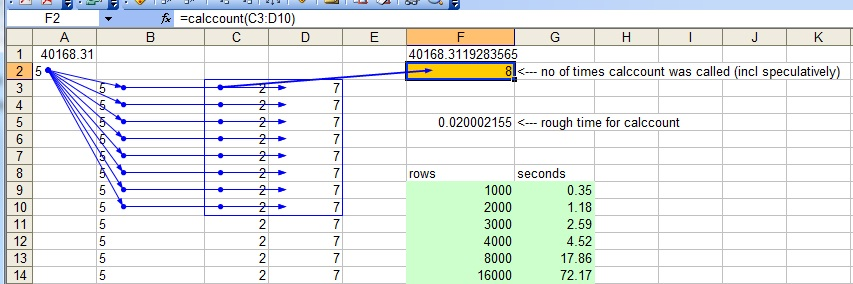benf.org :  excel stuff :  calcdep

An Addin (XLL) (Source here) and a sheet here, which seem to show that dependency discovery in excel is N^2 in time. (in the simple case below, in 2000, 2003, 'fixed' in 2007, but in a right deep tree, still expensive in 2007)What this is, is a very simple shallow (same distance from root to all cells in D, in the image) calculation tree, which is used as an input to an addin function "calccount".

If you have an 'R' type input to an addin function, you can be called with uncalculated inputs, at which point you have to return specifying that your inputs aren't sufficiently calced. Calccount shows the number of times it was 'speculatively' called, before it succeeds - you can see from the example sheet that it's called once per input row (in this case).

What's interesting about this example is that the time for calculation looks N^2 - even though we really wouldn't expect it to do so.

This is not related to the use of an R input, it has the same performance characteristics for a P input, but you don't get to see the number of speculative calls.

This is the case for Excel 2000, and Excel 2003. Excel 2007 doesn't have this cost in this case.

Costs:
rowsseconds
10000.35
20001.18
30002.59
40004.52
800017.86
1600072.17

For what it's worth, in the more complicated case where each row depends on the previous row, so we have a right deep calc tree, excel 2007 also exhibits this cost (to be honest, it's understandable!) You could imagine how you could get that in linear cost, but it's understandable that it doesn't.)

 Last updated 11/2009